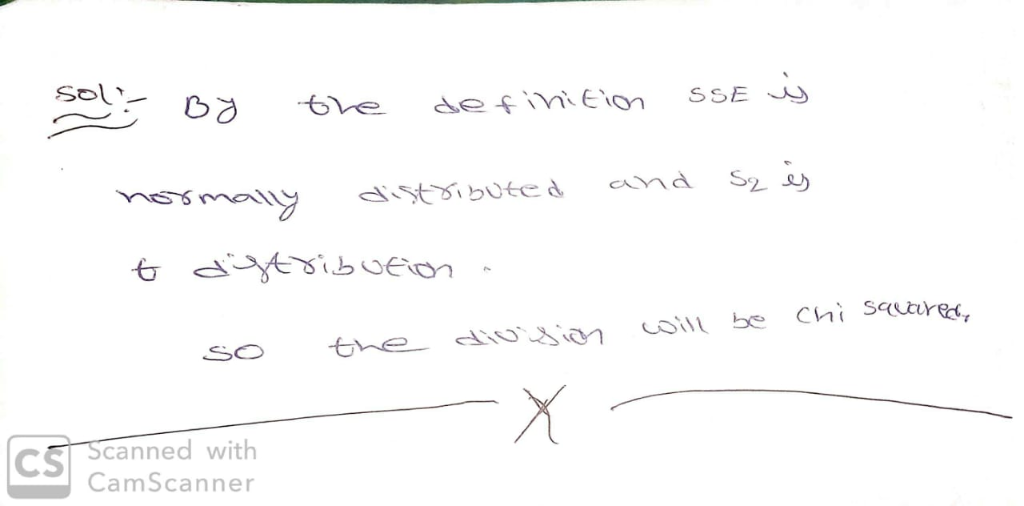# Suppose that independent samples of sizes n1, n2, . . . , nk are taken from each of k normally di...

Suppose that independent samples of sizes n1, n2, . . . , nk are taken from each of k normally distributed populations with means μ1,μ2, . . . , μk and common variances, all equal to σ 2. Let Yi j denote the j th observation from population i, for j = 1, 2, . . . , ni and i = 1, 2, . . . , k, and let n = n1 + n2 + ··· + nk . a Recall that SSE = bk i =1 (ni − 1)S2 i where S2 i = 1 ni − 1 bni j =1 (Yi j − Y i •)2. Argue that SSE/σ 2 has a χ 2 distribution with (n1 − 1) + (n2 − 1)+· · ·+(nk − 1) = n − k df. Argue that under the null hypothesis, H0 : μ1 = μ2 = ··· = μk all the Yi j ’s are independent, normally distributed random variables with the same mean and variance. Use Theorem 7.3 to argue further that, under the null hypothesis, Total SS = bk i =1 bni j =1 (Yi j − Y )2 is such that (Total SS)/σ 2 has a χ 2 distribution with n − 1 df. c In Section 13.3, we argued that SST is a function of only the sample means and that SSE is a function of only the sample variances. Hence, SST and SSE are independent. Recall that Total SS = SST + SSE. Use the results of Exercise 13.5 and parts (a) and (b) to show that, under the hypothesis H0 : μ1 = μ2 = ··· = μk, SST/σ 2 has a χ 2 distribution with k −1 df. d Use the results of parts (a)–(c) to argue that, under the hypothesis H0 : μ1 = μ2 = ··· = μk , F = MST/MSE has an F distribution with k − 1 and n − k numerator and denominator degrees of freedom, respectively.##### Add Answer of: Suppose that independent samples of sizes n1, n2, . . . , nk are taken from each of k normally di...
More Homework Help Questions Additional questions in this topic.